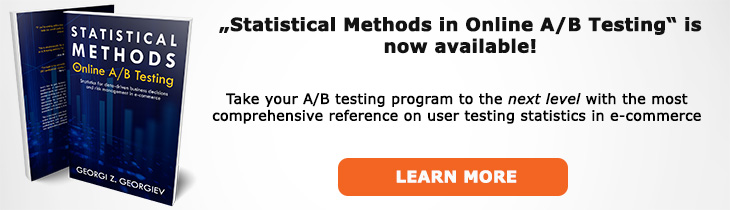# What does "Variance" mean?

Definition of Variance in the context of A/B testing (online controlled experiments).

## What is Variance?

Variance is a measure of dispersion of a set of values from the center of their distribution, a measure of central tendency. The higher the variance, the more spread the values are. The smaller it is, the more clustered they are.

Variance is calculated as the arithmetic mean (average) of the squared differences of each value from the arithmetic mean of the sample or population. The differences are squared so larger departures from the mean can be "punished" more severely. It also allows to treat departures in both direction the same (positive errors are treated the same as negative ones).

Since variance is a squared difference, it is hard to communicate in a meaningful manner most of the time. Usually what is communicated is the standard deviation: the square root of the variance, as it is expressed in the same unit as the data itself.

Understanding the variance of your data set is important in A/B testing since the more variance there is in your data, the longer you would need to test (larger sample sizes are required) to reach a specified level of confidence. When dealing with a binomial metric the variance is known analytically: the closer a proportion is to one of the extremes (0 or 1) the higher its variance is. When dealing with non-binomial metrics the population variance can be estimated through a sample.

## Related A/B Testing terms

Standard Deviation

Like this glossary entry? For an in-depth and comprehensive reading on A/B testing stats, check out the book "Statistical Methods in Online A/B Testing" by the author of this glossary, Georgi Georgiev.## Glossary Index by Letter

Select a letter to see all A/B testing terms starting with that letter or visit the Glossary homepage to see all.